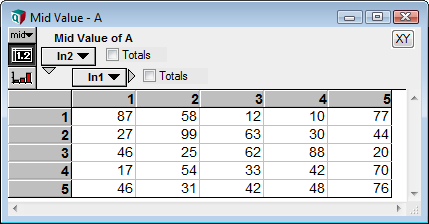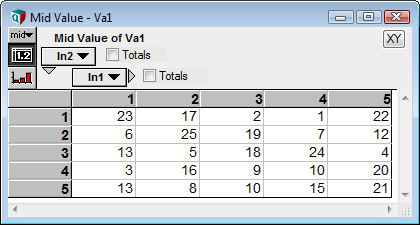# Flatten

new to Analytica 5.0

Release: 4.6  •  5.0  •  5.1  •  5.2  •  5.3  •  5.4  •  6.0  •  6.1  •  6.2  •  6.3  •  6.4

## Flatten(x, I..., resultIndex, condition)

Flattens the cells of a multi-dimensional array into a one-dimensional vector.

You should usually specify the indexes of «x» that you want to flatten over. For example, Flatten(x, In1, In2, In3) returns a one-dimensional array having the same values as the 3-dimensional array «x». The indexes listed first vary the slowest.

If you already have a result index, K for the final result, specify it by name using

Flatten(x, In1, In2, In3, resultIndex: K)

If you omit «resultIndex», a local index is created for you with the name .K. If your «resultIndex» is shorter than the number of cells, the flattened result is truncated, or if it is too long the result is null-padded.

Because a local index is created for the result, to define an index from the result of Flatten, you should surround it in CopyIndex(...).

If you omit «I» (the indexes to flatten), then all indexes of «x» are used, but you don't have explicit control over what order is used.

(New to Analytica 5.4) You can also specify an optional «condition» parameter to include only items in «x» that correspond to «condition» being true.

## Examples

### Applying a 1-D array-reducing function across multiple dimensions

Many array-reducing functions operate over one index and return a scalar result. For example, Median(a, I) computes the median of a data set along index I. Suppose you have a 2-D array, and you want the median value across both indexes. This is an example where you need to force a 1-D array-reducing function to operate over two or more indexes. This can be accomplished by flattening the array and then applying the array-reducing function.

A :=Local b:=Flatten(A, In1, In2) Do Median(b, b.K) → 46

This method easily generalizes to more than two indexes, e.g., for four indexes:

Local b:=Flatten(A, In1, In2, In3, In4) Do Median(b, b.K)

To apply the reducing function over all indexes of the array, without knowing the number of indexes in advance, use Repeated parameter forwarding, e.g.,

Local b:=Flatten(A, ...IndexesOf(A)) Do Median(b, b.K)

Many built-in array-reducing functions (including Sum, Max, Min, Product, Average) already accept multiple indexes, making this technique unnecessary. With these you can just list the indexes to reduce over, e.g., Sum(A, In1, In2, In3).

### Applying a 1-D transforming function across multiple dimensions

Situations sometime arise where you would like to apply an existing transforming function to a multidimensional array, such that the transformation should simultaneously operate over two or more indexes, but the existing function was created to operate over a single index. A transformation function is a function that takes an array as input, and produces an array having the same dimensions as the input.

One example is the Rank function, which operates over a single index. Suppose you want to apply it over 2 or more dimensions. You can do this by flattening the input array, applying Rank to the flattened 1-D result and then unflattening the result.

A :=Local b := Flatten( A, In1, In2 ) Do Unflatten( Rank( b, b.K ), b.K, In1, In2 )Notice that the smallest cell in the input has a rank of 1, and the largest value has a rank of 25.

The above example does a 2-D Rank calculation, and the same idea could be used for a 3-D (and so on) rank, e.g.,

Local b := Flatten( A, In1, In2, In3 ) Do Unflatten( Rank( b, b.K ), b.K, In1, In2, In3 )

This can be generalized to a User-Defined Function that works with any number of dimensions, by using Repeated parameter forwarding as follows.

Function RankMultiD( a : Array[I] ; I : ... index )
Definition: Local b := Flatten( a, ...I ) Do Unflatten( Rank( b, b.K ), b.K, ...I )

You can use this function to compute Rank over three dimensions as

RankMultiD( A, In1, In2, In3 )

Or, you can use this to compute the rank over all indexes of A, without knowing in advance what they are, using Repeated parameter forwarding as

RankMultiD( A, ...IndexesOf(A) )

### Customizing «resultIndex» labels

When you let the function create a local «resultIndex» for you, it defines the index elements as 1 to n. When you want the index to consist of some combination of the original source indexes, you should call Flatten twice, the first time to create the custom index labels, and the second time to flatten the values.

Index Year := 2017..2030
Index Month := DatePart(MakeDate(2017,1..12),"MMM") { This is ['Jan', 'Feb', ..., 'Dec'] }
Index BiMonthDay := [1,15]

We want to flatten Forecasted_price, but we want the index labels to be ['2017-Jan-1', '2017-Jan-15', '2017-Feb-1',...] and not just 1..24336. Note that this also gives you an opportunity to select the name for the local index. The use of CopyIndex here is unnecessary when defining a local index, but would be required in the Definition of a global index.

 

LocalIndex FlatDate := CopyIndex( Flatten(Year & '-' & Month & '-' & BiMonthDay, Year, Month, ByMonthDay) ); Flatten( Forecasted_price, Year, Month, BiMonthDay, K )